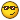Fibonacci component for hal, houston we have a problem

30 Oct 2018 00:33 #119632 by Grotius
I was watching Youtube and saw Elon Musk of Tesla, Space ex, etc Talking about AI.
Then he warned about AI (arteficial intelligence).

So a few minutes after the film i had an idea to calculate a special number in C.
In fact calculate a special model that is related tho mother Earth and put it in a realtime Hal component.

The output is amazing. I had to make an enable pin for the function, otherwise it go's too fast.
The prime value is found so fast. Wow man.
en.wikipedia.org/wiki/2,147,483,647#Barlow's_prediction

The Fibonacci Model is found even so fast :
ascensionglossary.com/images/d/de/Fibonaccispiral.jpg

I will look to print exponential value's to the maximum possibility.

For those who like this, here is the C component :
component fibonacci"Fibonacci";

description
"""

Fibonacci Component
https://en.wikipedia.org/wiki/Fibonacci_number

Improvements :
This component can be related to time and space.

Practical usage :
The output can be adapted to external offset branche to visualise the Fibonacci effect.
The output can be inversed when expanding the code.

""";

author "Grotius CNC Machines, Oktober 2018";

option singleton yes;
//option userspace yes;

//Input hal connection :

//Output hal connection :
pin in bit Enable;             //Trigger this rapid function..
variable float A;                //u32 is only positive to 4294967295
variable float B;                //s32 values = integer numbers -2147483648 to 2147483647

pin out u32 C_old;             //
pin out u32 C_new;             //
pin out u32 C_sum;             //
pin out u32 Fibonacci_value    "Same pin as C_sum";

variable double Time;          //timer
variable int Counter;

function _;

;;

#include "rtapi.h"		/* RTAPI realtime OS API */
#include "rtapi_app.h"		/* RTAPI realtime module decls */
#include "rtapi_string.h"
#include "hal.h"		/* HAL public API decls */
#include "stdio.h"
#include "rtapi_math.h"

typedef enum { INIT, POSITIVE_LOOP, STOP} state_T;  // To be expand with negative loop.
state_T state = INIT;

FUNCTION(_) {

switch(state){

case INIT:

//Visual Fibonacci calculation setup :
//Line_-6.    -8 - 5 = -13
//Line_-5.    -5 - 3 = -8
//Line_-4.    -3 - 2 = -5
//Line_-3.    -2 - 1 = -3
//Line_-2.    -1 - 1 = -2
//Line_-1.     0 - 1 = -1
//Line_0.      0 = 0
//Line_1.      0(A)     + 1(B) = 1(C_old)  --> Begin startup loop
//Line_2.      1(C_old) + 1(B) = 2(C_new)                         --> (C_old)
//Line_3.                        3(C_sum)  --> End startup loop   --> (C_new)   --> (C_old)
//Line_4.                        5(C)                             --> (C_sum)   --> (C_new)  --> (C_old)
//Line_5.                        8(C)                                           --> (C_sum)  --> (C_new)
//Line_6.                       13(C)                                                        --> (C_sum)

C_old = 0;
C_new = 0;
C_sum = 0;
Counter = 0;

//INIT
A = 0;
B = 1;
Time = 0; //Not used today.

//Begin Startup loop.
C_old = A + B;          // = 0 + 1 = 1
C_new = C_old + B;      // = 1 + 1 = 2
C_sum = C_old + C_new;  // = 3
//End startup loop, C_sum has the first calculated output value. 1 + 2 = 3

//Start positive loop.
state = POSITIVE_LOOP;
break;

case POSITIVE_LOOP:

if(Enable){
//Begin Fibonacci loop.
C_old = C_new;                   //Step up 2
C_new = C_sum;                   //Step up 3
C_sum = C_old + C_new;           //New output value, 2+3=5 next, next 5+3=8, ..
printf("%d\n", C_sum);
Fibonacci_value = C_sum;
if(C_sum = 2147483647){state = STOP;}
}

//if(Fibonacci_value > 9000000000000000000){printf("We are in singulatiry mode");}

if(!Enable){
state = INIT;
// Start from zero again ...
}

break;

case STOP:

if(Enable){
printf("C_sum found at = 2147483647");
}

if(!Enable){
state = INIT;
}

//https://en.wikipedia.org/wiki/2,147,483,647#Barlow's_prediction

break;

}

}

The following user(s) said Thank You: tommylight

31 Oct 2018 21:32 - 31 Oct 2018 21:53 #119730 by Grotius
Update :

I think within the Fibonacci effect i can use a circle movement to visualize the effect in X,Y. (Time)
In Z what is not visual actually in the picture, we can use the visual output of (Space).
Opa's example in External offset branche is a basic circle
that we can expand to visualize this special function.

I need to do some scaling's to go back in time. Realtime is to fast for this function, and go's out of range for any pc at the moment,
the current code's are not written for huge digit's. The computer code an sich is not the problem. It's that i need to change
coding to very large number's, include to find and calculate back prime numbers in sequence, in this way i can find a new record.
In this way the Bitcoin will be useless in short time. When using large number's
everything will be solved very quicly. My pc is limited to a value ca. 9900000000.... at 64 bit, So that is a certain problem i want to solve within a very fast real time C component. ( i hope 10 lines of core looping code ). Kali can't do this with current kernel.for Linuxcnc real time, It's an easy job. So don't forget the power of real time Linuxcnc with custom made user gui. The power is huge.
When you startup your pc for the first time. They say in terminal : use your power for good purposes..... So i agree.

If i do it good. The largest exponential number calculation wil be a text file as long as i will.
So i have to think about a new type of value storing. String format in excel based format, then the output quilt be
seqeunced in different grades. I think this idea is growing.
Last edit: 31 Oct 2018 21:53 by Grotius.
The following user(s) said Thank You: tommylight